# Domination in plane triangulations

 Importance: Medium ✭✭
 Author(s): Matheson, Lesley R. Tarjan, Robert E.
 Subject: Graph Theory » Topological Graph Theory
 Keywords: coloring domination multigrid planar graph triangulation
 Posted by: mdevos on: May 4th, 2009
Conjecture   Every sufficiently large plane triangulationhas a dominating set of size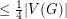.

Motivated by some problems in multigrid computations, Matheson and Tarjan [MT] considered the problem of finding small dominating sets in plane triangulations. They proved that every such graphhas a dominating set of sizeand posed the above question.

The Octahedron is a triangulation with 6 vertices for which every dominating set has size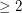, so no constant better thancan be achieved in general. However, it appears that one can do better for larger graphs. The most extreme examples here (also from [MT]) are constructed as follows: Start withdisjoint copies ofembedded in the plane, and then add edges to complete this graph to a triangulation (with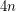vertices). Now each of the original copies ofhas an inner vertex which has degree 3 in the final graph, and in order to cover it, one must take at least one vertex from this. It follows that every dominating set has size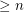.

Since the Matheson-Tarjan proof is short and instructive, we sketch it here. In fact, we shall prove (as they did) the stronger statement that every near-triangulation (a graph embedded in the plane with all finite faces of size three) has a (possibly improper) 3-coloring so that each color class is a dominating set and so that the subgraph induced by those vertices incident with the infinite face is properly colored. This stronger fact we prove by induction. If the infinite face is not bounded by a cycle or the infinite face is bounded by a cycle which has a chord, then the graph may be written as the union of two near-triangulationswhere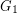andeither share one vertex or two adjacent vertices and one edge. In either case, the result follows by applying induction toand. Otherwise, choose a vertexon the infinite face, deleteand apply induction. Since the neighbors ofare all on the infinite face, and do not form an independent set, there are at least two colors, sayand, which appear on the neighbors of. Now givingthe colorgives a solution.

## Bibliography

[MT] L. R. Matheson, R. E. Tarjan, Dominating sets in planar graphs. European J. Combin. 17 (1996), no. 6, 565--568. MathSciNet

* indicates original appearance(s) of problem.